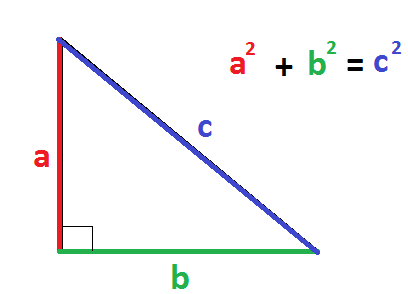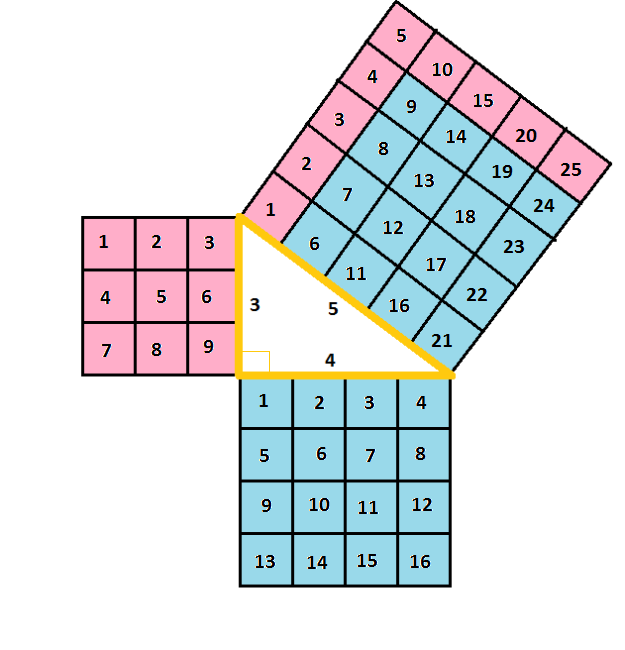# Pythagorean Theorem Proof: Lesson for Kids

Instructor: Allison Petrovic

Allison has experience teaching high school and college mathematics and has a master's degree in mathematics education.

Did you know that we can use art and math together? In this lesson, we will use one picture to prove the famous Pythagorean Theorem! Join me to see how this Pythagorean Theorem Proof works with art.

## What is the Pythagorean Theorem?

Let's have some fun and combine art with math! But, before we can begin, let's review a couple of terms. The Pythagorean Theorem says that for right triangles, the sum of the squares of the leg measurements is equal to the hypotenuse measurement squared. The legs are the two shorter sides of a right triangle. The hypotenuse is the longest side of a right triangle. Let's look at the 'Definition of Pythagorean Theorem' picture to get a better understanding. Letter a represents the length of one leg. Letter b represents the length of the other leg, and letter c is the length of the hypotenuse!## Here's the Proof!

Look at the 'Proof of Pythagorean Theorem' image which shows a right triangle outlined in orange. The two legs are 3 units and 4 units. The hypotenuse is 5 units. Each side of the triangle has a square that goes along with it. So the leg that is 3 units has a 3 x 3 square. This square is pink. The leg that is 4 units has a 4 x 4 square. This square is blue. The hypotenuse, which is 5 units, has a 5 x 5 square. This square is pink and blue.## Art Proves the Pythagorean Theorem

This picture is showing us the Pythagorean Theorem! Let's break it down. First, we need to square each of the legs. For the leg that is 3 units, we can square 3 (32) and get 3 x 3 = 9. In the picture, the pink square is made up of 9 units. For the leg that is 4 units, we can square 4 (42) and get 4 x 4 = 16. In this picture, the blue square has 16 units! If we add those two squares together, we should get the square of the hypotenuse according to the Pythagorean Theorem! Adding the two squares together, we get 9 + 16 = 25 square units!

To unlock this lesson you must be a Study.com Member.

### Register to view this lesson

Are you a student or a teacher?

#### See for yourself why 30 million people use Study.com

##### Become a Study.com member and start learning now.
Back
What teachers are saying about Study.com

### Earning College Credit

Did you know… We have over 200 college courses that prepare you to earn credit by exam that is accepted by over 1,500 colleges and universities. You can test out of the first two years of college and save thousands off your degree. Anyone can earn credit-by-exam regardless of age or education level.